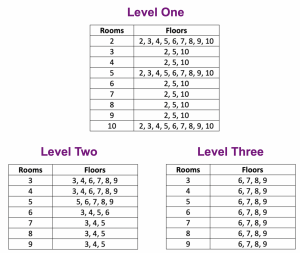# Splitting Arrays

In last month’s Construct a Building post, I presented any array model in which students construct the rooms and floors of a building as a way of representing multiplication. Now I’d like to follow up with a similar array model that allows students to take a problem they don’t know, like 8 × 7, and break it into two simpler problems they do know, like 5 × 7 and 3 × 7.

There are three levels to the game below (and here), each on its own page. For each multiplication problem, you can model it with the interactive array. You can adjust the dimensions of the array below by dragging the point at its top-right corner. You can split the array into two smaller arrays by dragging the slider along the array’s left edge.

There are 10 problems per game, with a maximum score of 100 points. Missing a problem and trying again reduces the value of a correct answer by 2 points. Problems are presented randomly in each level, with level one focusing on multiples of 2, 5, and 10 and levels two and three progressing to other multiples. The tables at the end of this post list the possible problems for each level.

If you like this game, you should check out Bunny Times, a multiplication game that we developed for the Dynamic Number project. It also focuses on arrays, and features adorable artwork from a Disney animator.